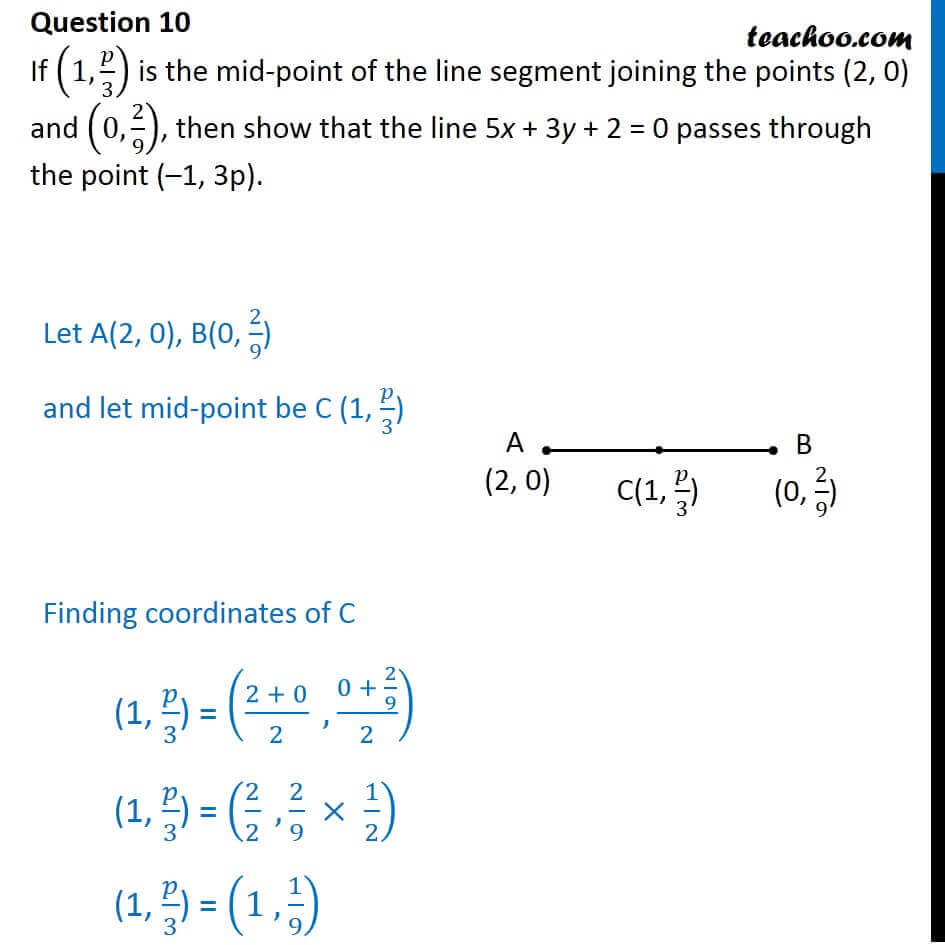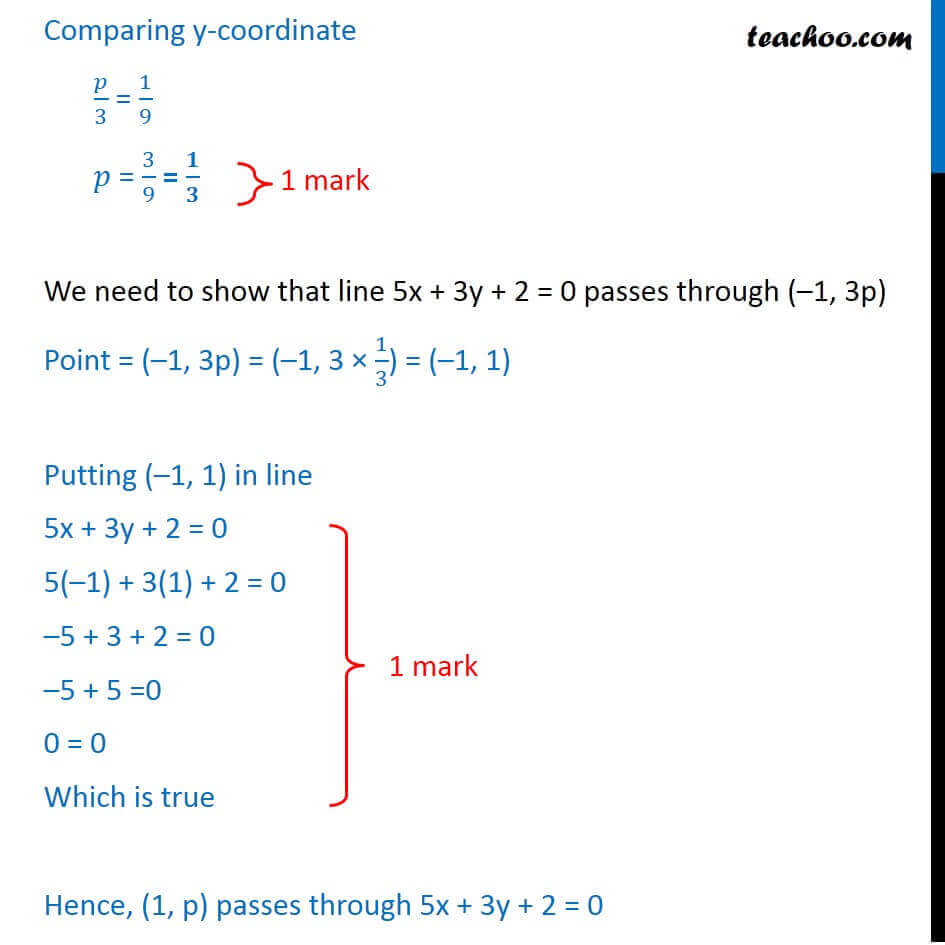CBSE Class 10 Sample Paper for 2018 Boards

Class 10
Solutions of Sample Papers for Class 10 Boards

### If (1, p/3) is the mid-point of the line segment joining the points (2, 0) and (0, 2/9), then show that the line 5 x + 3 y + 2 = 0 passes through the point (–1, 3p).

This is a question of CBSE Sample Paper - Class 10 - 2017/18.Learn in your speed, with individual attention - Teachoo Maths 1-on-1 Class

### Transcript

Question 10 If (1, /3) is the mid-point of the line segment joining the points (2, 0) and (0, 2/9), then show that the line 5x + 3y + 2 = 0 passes through the point ( 1, 3p). Let A(2, 0), B(0, 2/9) and let mid-point be C (1, /3) Finding coordinates of C (1, /3) = ((2 + 0)/2 , (0 + 2/9)/2) (1, /3) = (2/2 ,2/9 1/2) (1, /3) = (1 ,1/9) Comparing y-coordinate /3 = 1/9 = 3/9 = / We need to show that line 5x + 3y + 2 = 0 passes through ( 1, 3p) Point = ( 1, 3p) = ( 1, 3 1/3) = ( 1, 1) Putting ( 1, 1) in line 5x + 3y + 2 = 0 5( 1) + 3(1) + 2 = 0 5 + 3 + 2 = 0 5 + 5 =0 0 = 0 Which is true Hence, (1, p) passes through 5x + 3y + 2 = 0